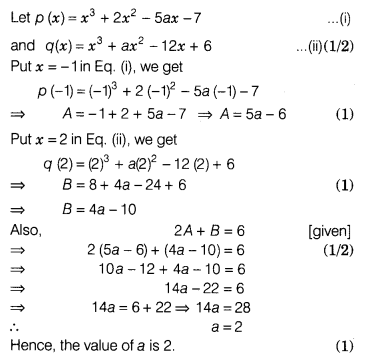# If A and B are the remainders when the polynomials x2, + 2x2 - 5ax-7

If A and B are the remainders when the polynomials x2, + 2x2 - 5ax-7 and x3 + ax2 - I2x + 6 are divided by (x +1) and (x - 2) respectively, and 2A + B = 6, then find the value of a.